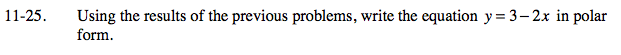Home > PC > Chapter 11 > Lesson 11.1.2 > Problem11-25

11-25.

Using the results of the previous problems, write the equation y = 3 − 2x in polar form. Homework Help ✎Use x = r cos(θ) and y = r sin(θ).
Be sure to write your answer in r = form.